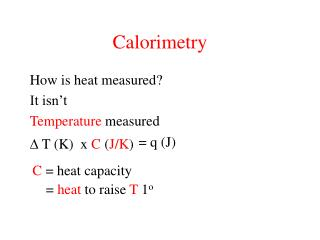DownloadDownload PresentationCalorimetry

# Calorimetry

Download Presentation## Calorimetry

- - - - - - - - - - - - - - - - - - - - - - - - - - - E N D - - - - - - - - - - - - - - - - - - - - - - - - - - -
##### Presentation Transcript

1. Calorimetry How is heat measured? It isn’t Temperature measured = q (J)  T (K) x C (J/K) C = heat capacity = heat to raise T 1o

2. heat capacity specific heat capacity J / K g J / K molar heat capacity mol q (J) = x T (K) x mass (g) C (J / K g) qsystem = - qsurroundings (reaction) (thermometer)

3. heat capacity K.E.translational - raise T of system heat + K.E.vibrational + P.E. • K.E.rotational compare: water Helium He

4. heat capacities substance specific m.w. molar heat capacity (g/mol) heat capacity (J / K g) (J / K mol) 26.98 24.0 Al (s) 0.89 24.8 Fe (s) 0.44 55.85 H2O(s) 36.5 75.2 H2O(l) 133.0 CCl4(l)

5. Calorimetry 1. Measure T (Tfinal - Tinitial) 2. Convert to q q (J) T (K) x C (J/K g) x mass (g) =

6. Heat transfer experiments 150 mL Tf q = C x T x mass 50 mL 100o 100 mL 25o q1 = (4.184 J/oC g) x (Tf - 100) x (50 g) q2 = (4.184 J/oC g) x (Tf - 25) x (100 g) q1 = - q2 (Tf - 100) x (50) = - (Tf - 25) x (100) Tf = 50o C

7. Calorimetry 1. Measure T (Tfinal - Tinitial) 2. Convert to q q (J) T (K) x C (J/K g) x mass (g) = q is a path function E = q + w E - w E + PextV q = =

8. q = E + PextV H Enthalpy H  E + PV H = E + PV H = E + PV +VP At constant P, P = 0 H = E + PV q = E + PV = H q p

9. Enthalpy H  E + PV Extensive State function Hrxn = qrxn = qsystem = - qsurroundings (reaction) (thermometer)

10. Enthalpy of reaction Hrxn= qrxn coffee cup calorimeter 10.5 g KBr in 125g water at 24o KBr(s)  K+ (aq) + Br-(aq) Tf = 21o Calculate Hrxn qsystem = - qsurroundings = Hrxn

11. Hrxn qsystem = - qsurroundings = Hrxn T x mass qsurroundings = C x (4.184 J/goC) (21 - 24oC) (10.5 g + 125 g) qsurroundings = = -1756 J = +1756 J = Hrxn qsystem = - qsurroundings H is extensive Hrxn = 1756 J = 167 J/g = 19873 J/mol 10.5 g KBr

12. E = q + w E = q - PV At constant V, E = qv Bomb calorimeter qrxn = qsystem = -qcalorimeter T (oC) qcalorimeter = C (J / oC) x

13. Constant Volume calorimetry 2Fe (s) + 3/2 O2 (g)  Fe2O3 (s) 11.2 g Fe(s), 1 atm O2 Ccalorimeter = 2.58 kJ/oC Tcalorimeter = + 31.9 oC -qcalorimeter qrxn = = Erxn = - (2.58 kJ/oC) (31.9oC) Erxn / 0.1 mol Fe2O3 = - 82.2 kJ = - 822 kJ/mol Fe2O3

14. qv v.s. qp qp = H H = E + PV qv = E = E + nRT H = E + PV if n = 0 H = E 2Fe (s) + 3/2 O2 (g)  Fe2O3 (s) n = (0 - 3/2) = - 3/2 H = (- 3/2)(8.314 x 10-3 kJ)(298) - 822 kJ/mol + H = -826 kJ/mol